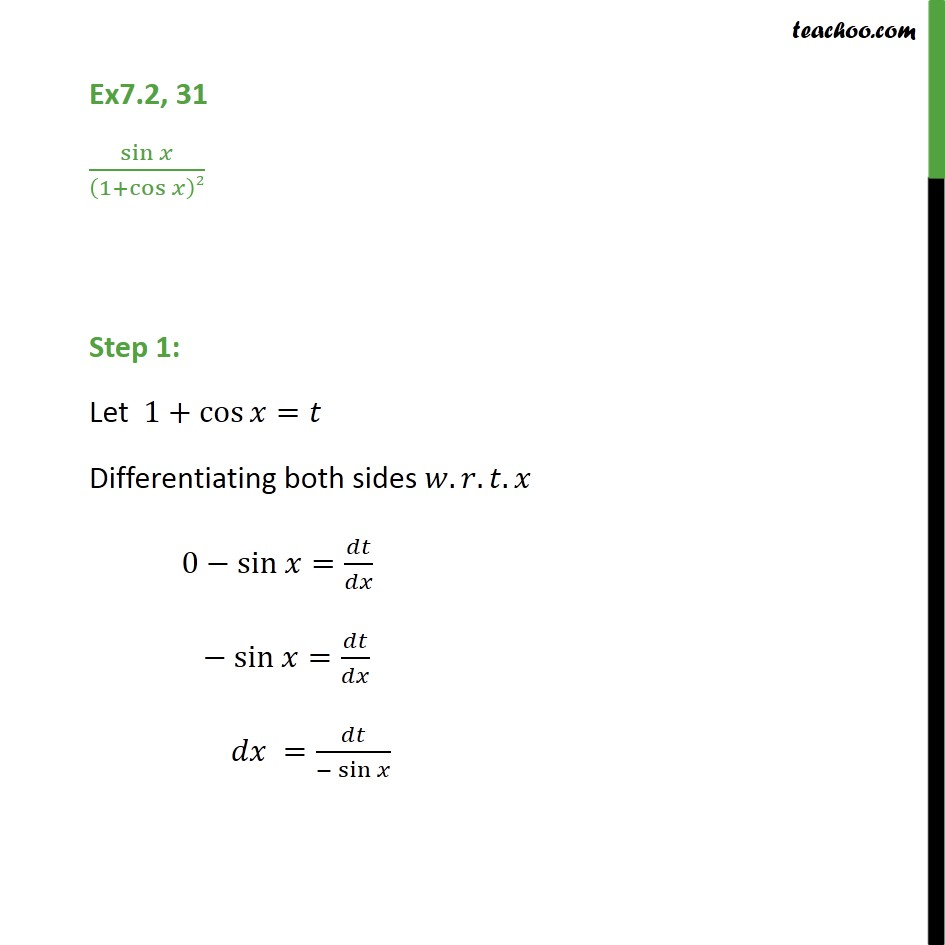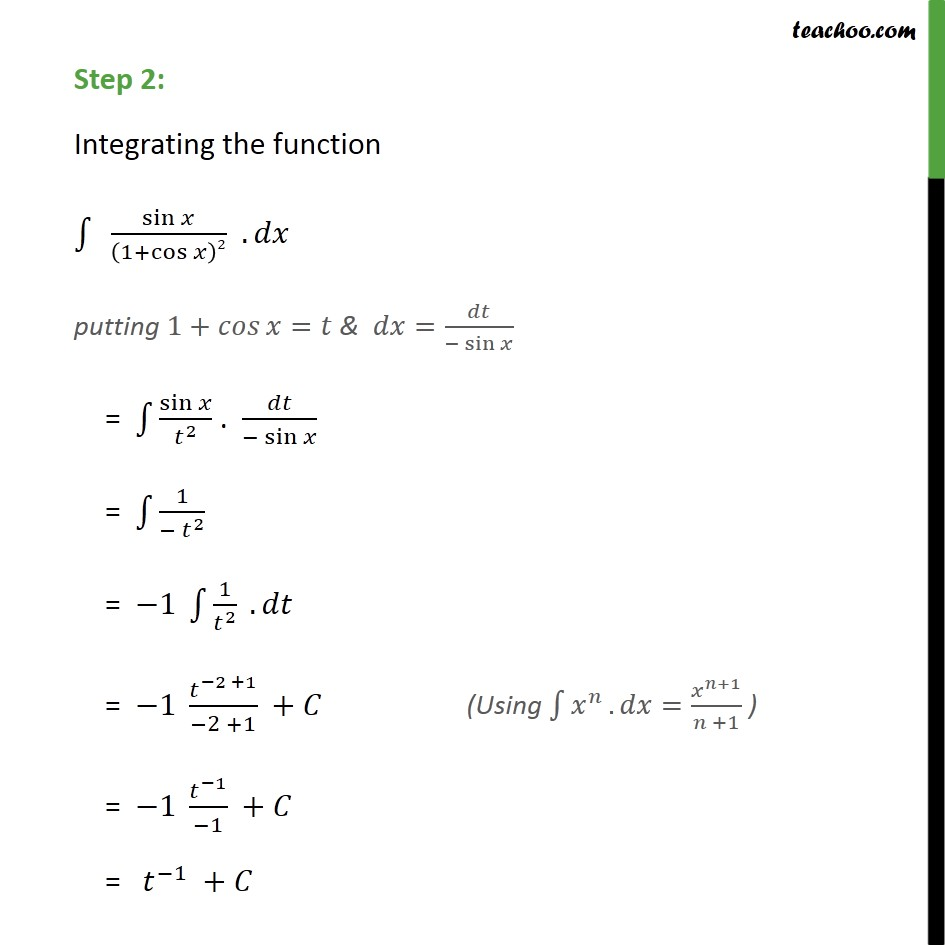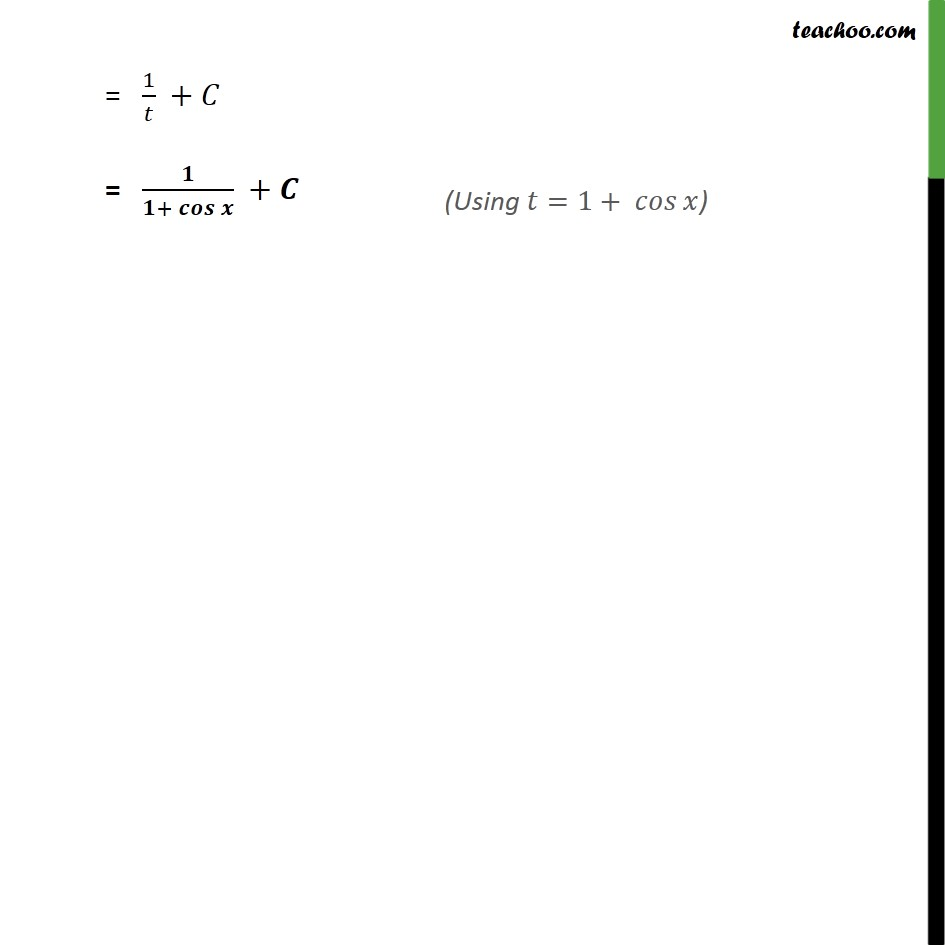1. Chapter 7 Class 12 Integrals (Term 2)
2. Concept wise
3. Integration by substitution - Trignometric - Normal

Transcript

Ex7.2, 31 sin﷮𝑥﷯﷮ 1+ cos﷮𝑥﷯﷯2﷯ Step 1: Let 1+ cos﷮𝑥﷯=𝑡 Differentiating both sides 𝑤.𝑟.𝑡.𝑥 0−sin 𝑥= 𝑑𝑡﷮𝑑𝑥﷯ − sin 𝑥= 𝑑𝑡﷮𝑑𝑥﷯ 𝑑𝑥 = 𝑑𝑡﷮− sin 𝑥﷯ Step 2: Integrating the function ﷮﷮ sin﷮𝑥﷯﷮ 1+ cos﷮𝑥﷯﷯2﷯ ﷯. 𝑑𝑥 putting 1+ 𝑐𝑜𝑠﷮𝑥﷯=𝑡 & 𝑑𝑥= 𝑑𝑡﷮− sin 𝑥﷯ = ﷮﷮ sin﷮𝑥﷯﷮ 𝑡﷮2﷯﷯﷯ . 𝑑𝑡﷮− sin 𝑥﷯ = ﷮﷮ 1﷮− 𝑡﷮2﷯﷯﷯ = −1 ﷮﷮ 1﷮ 𝑡﷮2﷯﷯﷯ . 𝑑𝑡 = −1 𝑡﷮−2 +1﷯﷮−2 +1﷯ +𝐶 = −1 𝑡﷮−1﷯﷮−1﷯ +𝐶 = 𝑡﷮−1﷯ +𝐶 = 1﷮𝑡﷯ +𝐶 = 𝟏﷮𝟏+ 𝒄𝒐𝒔﷮𝒙﷯﷯ +𝑪